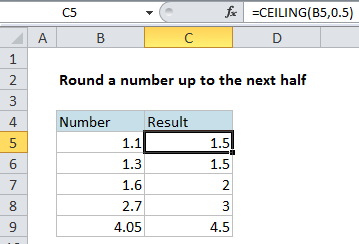## Excel Office

Excel How Tos, Tutorials, Tips & Tricks, Shortcuts

# Round a number up to next half in Excel

This tutorials shows how to Round a number up to next half in Excel.

If you need to round a number up to the next half, you can use the CEILING function, which always rounds up based on a supplied multiple.

## Formula

`=CEILING(number,0.5)`Note that MROUND also rounds based on a supplied multiple, but it always rounds to the nearest multiple.

Also See:   Round a number to nearest multiple in Excel

## Explanation

In the example, the formula shown is:

`=CEILING(B5,0.5)`

Even though the value in B5 is 1.1, CEILING rounds up to the next multiple of .5, which is 1.5